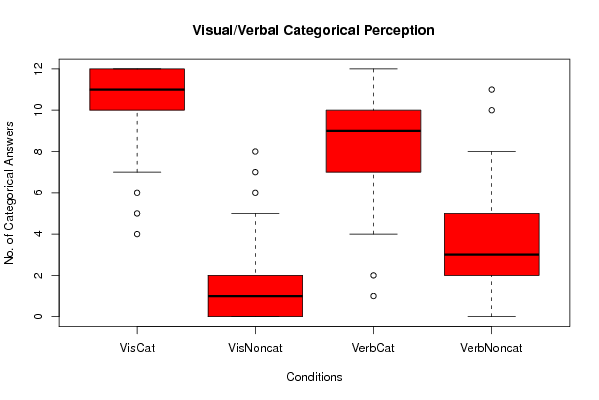## Free Statistics

of Irreproducible Research!

Author's title
Author*The author of this computation has been verified*
R Software ModuleIan.Hollidayrwasp_Tests to Compare Two Means.wasp
Title produced by softwareAston University Statistical Software
Date of computationMon, 16 Nov 2009 15:01:38 -0700
Cite this page as followsStatistical Computations at FreeStatistics.org, Office for Research Development and Education, URL https://freestatistics.org/blog/index.php?v=date/2009/Nov/16/t1258408939rnp50wnzrnrwpnn.htm/, Retrieved Fri, 09 Jun 2023 18:14:44 +0000
Statistical Computations at FreeStatistics.org, Office for Research Development and Education, URL https://freestatistics.org/blog/index.php?pk=57356, Retrieved Fri, 09 Jun 2023 18:14:44 +0000
QR Codes:Original text written by user:
IsPrivate?No (this computation is public)
User-defined keywords
Estimated Impact225
Family? (F = Feedback message, R = changed R code, M = changed R Module, P = changed Parameters, D = changed Data)
-     [Aston University Statistical Software] [Test of Two Means] [2009-11-10 17:16:17] [98fd0e87c3eb04e0cc2efde01dbafab6]
- R P   [Aston University Statistical Software] [Compare Two Means] [2009-11-11 08:09:40] [74be16979710d4c4e7c6647856088456]
-    D    [Aston University Statistical Software] [PY2236 Week 6 dat...] [2009-11-11 12:53:10] [74be16979710d4c4e7c6647856088456]
-   PD        [Aston University Statistical Software] [Comparison 2] [2009-11-16 22:01:38] [8b088281e1eac39fda503d484618b84c] [Current]
Feedback Forum

Post a new message
Dataseries X:
4	8	7	5
5	7	4	8
6	6	2	10
7	5	5	7
7	5	8	4
8	4	8	4
8	4	7	5
9	3	10	2
9	3	8	4
9	3	8	4
9	3	10	2
9	3	11	1
10	2	7	5
10	2	9	3
10	2	9	3
10	2	11	1
10	2	4	8
10	2	7	5
10	2	6	6
10	2	9	3
10	2	8	4
10	2	1	11
10	2	6	6
10	2	7	5
10	2	10	2
10	2	6	6
10	2	6	6
10	2	9	3
10	2	12	0
10	2	7	5
11	1	11	1
11	1	10	2
11	1	9	3
11	1	9	3
11	1	7	5
11	1	9	3
11	1	10	2
11	1	8	4
11	1	11	1
11	1	9	3
11	1	8	4
11	1	11	1
11	1	8	4
11	1	8	4
11	1	11	1
11	1	4	8
11	1	11	1
11	1	10	2
11	1	7	5
11	1	11	1
11	1	7	5
11	1	12	0
12	0	10	2
12	0	10	2
12	0	9	3
12	0	7	5
12	0	6	6
12	0	10	2
12	0	7	5
12	0	10	2
12	0	11	1
12	0	10	2
12	0	7	5
12	0	11	1
12	0	9	3
12	0	8	4
12	0	10	2
12	0	9	3
12	0	8	4
12	0	8	4
12	0	7	5
12	0	10	2
12	0	9	3
12	0	7	5
12	0	11	1
12	0	9	3
12	0	9	3
12	0	8	4
12	0	9	3
12	0	11	1
12	0	8	4
12	0	10	2
12	0	7	5
12	0	11	1
12	0	11	1
12	0	9	3


 Summary of computational transaction Raw Input view raw input (R code) Raw Output view raw output of R engine Computing time 1 seconds R Server 'Gwilym Jenkins' @ 72.249.127.135

\begin{tabular}{lllllllll}
\hline
Summary of computational transaction \tabularnewline
Raw Input & view raw input (R code)  \tabularnewline
Raw Output & view raw output of R engine  \tabularnewline
Computing time & 1 seconds \tabularnewline
R Server & 'Gwilym Jenkins' @ 72.249.127.135 \tabularnewline
\hline
\end{tabular}
%Source: https://freestatistics.org/blog/index.php?pk=57356&T=0

[TABLE]
[ROW][C]Summary of computational transaction[/C][/ROW]
[ROW][C]Raw Input[/C][C]view raw input (R code) [/C][/ROW]
[ROW][C]Raw Output[/C][C]view raw output of R engine [/C][/ROW]
[ROW][C]Computing time[/C][C]1 seconds[/C][/ROW]
[ROW][C]R Server[/C][C]'Gwilym Jenkins' @ 72.249.127.135[/C][/ROW]
[/TABLE]
Source: https://freestatistics.org/blog/index.php?pk=57356&T=0

Globally Unique Identifier (entire table): ba.freestatistics.org/blog/index.php?pk=57356&T=0

As an alternative you can also use a QR Code:

The GUIDs for individual cells are displayed in the table below:

 Summary of computational transaction Raw Input view raw input (R code) Raw Output view raw output of R engine Computing time 1 seconds R Server 'Gwilym Jenkins' @ 72.249.127.135

 Students T-Test Statistic P-value Students T-Test 9.80827 0

\begin{tabular}{lllllllll}
\hline
Students T-Test \tabularnewline
& Statistic & P-value \tabularnewline
Students T-Test & 9.80827 & 0 \tabularnewline
\hline
\end{tabular}
%Source: https://freestatistics.org/blog/index.php?pk=57356&T=1

[TABLE]
[ROW][C]Students T-Test[/C][/ROW]
[ROW][C][/C][C]Statistic[/C][C]P-value[/C][/ROW]
[ROW][C]Students T-Test[/C][C]9.80827[/C][C]0[/C][/ROW]
[/TABLE]
Source: https://freestatistics.org/blog/index.php?pk=57356&T=1

Globally Unique Identifier (entire table): ba.freestatistics.org/blog/index.php?pk=57356&T=1

As an alternative you can also use a QR Code:

The GUIDs for individual cells are displayed in the table below:

 Students T-Test Statistic P-value Students T-Test 9.80827 0PNG link Postscript link PDF link

Parameters (Session):
par1 = two.sided ; par2 = 1 ; par3 = 3 ; par4 = Students T-Test ; par5 = paired ; par6 = 0.0 ; par8 = FALSE ;
Parameters (R input):
par1 = two.sided ; par2 = 1 ; par3 = 3 ; par4 = Students T-Test ; par5 = paired ; par6 = 0.0 ; par7 = ; par8 = FALSE ;
R code (references can be found in the software module):
par2 <- as.numeric(par2)par3 <- as.numeric(par3)par4 <- as.character(par4)par5 <- as.character(par5)par6 <- as.numeric(par6)if ( par5 == 'unpaired') paired <- FALSE else paired <- TRUEx <- t(y)bitmap(file='test1.png')(r<-boxplot(x ,xlab=xlab,ylab=ylab,main=main,notch=FALSE,col=2))dev.off()load(file='createtable')if( par4 == 'Wilcoxon-Mann_Whitney'){a<-table.start()a <- table.row.start(a)a <- table.element(a,'Wilcoxon Test',3,TRUE)a <- table.row.end(a)a <- table.row.start(a)a <- table.element(a,'',1,TRUE)a <- table.element(a,'Statistic',1,TRUE)a <- table.element(a,'P-value',1,TRUE)a <- table.row.end(a)W <- wilcox.test(x[,par2],x[,par3],alternative=par1, paired = paired)a<-table.row.start(a)a<-table.element(a,'Wilcoxon Test',1,TRUE)a<-table.element(a,W$statistic[])a<-table.element(a,round(W$p.value, digits=5) )a<-table.row.end(a)a<-table.end(a)table.save(a,file='mytable.tab')}if( par4 == 'Students T-Test'){a<-table.start()a <- table.row.start(a)a <- table.element(a,'Students T-Test',3,TRUE)a <- table.row.end(a)a <- table.row.start(a)a <- table.element(a,'',1,TRUE)a <- table.element(a,'Statistic',1,TRUE)a <- table.element(a,'P-value',1,TRUE)a <- table.row.end(a)T <- t.test(x[,par2],x[,par3],alternative=par1, paired=paired, mu=par6)a<-table.row.start(a)a<-table.element(a,'Students T-Test',1,TRUE)a<-table.element(a,round(T$statistic[], digits=5) )a<-table.element(a,round(T$p.value, digits=5) )a<-table.row.end(a)a<-table.end(a)table.save(a,file='mytable.tab')}